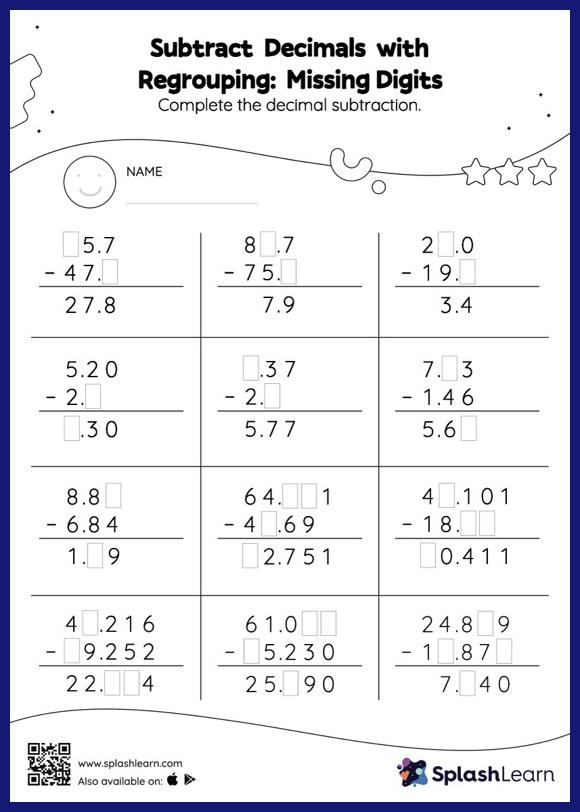# Subtract Decimals with Regrouping: Missing Digits Worksheet

Home > Subtract Decimals with Regrouping: Missing DigitsGive your little one some practice building subtraction skills with this subtract decimals with regrouping worksheet. Students use the relationship between addition and subtraction to find the missing number while subtracting decimals. To reach the answer, they must also regroup the numbers in subtract decimals with regrouping worksheet using the relationships between ones, tenths, hundredths, thousandths, etc.